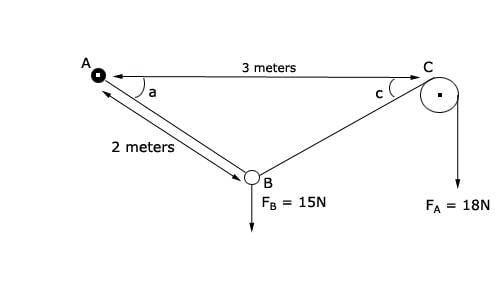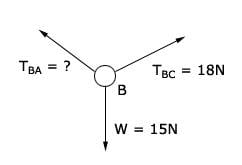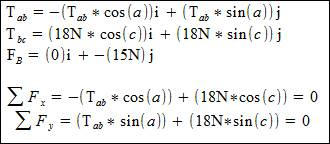# Forces in equilibrium. Tension, ring, pulley and lots of string [With Picture!]

## Homework Statement

To make it easier on the people who will try to help me, I've scanned the problem:We are interested in finding the following variables:
Angles a and c
The tension between A and B (TAB)

## Homework Equations

The pulley is a "perfect pulley" no friction
The system is at equilibrium
We consider on side A that the string is attached to the wall, or a hook, it's fixed.
All three cords are attached to a ring situated at position B
There is nothing between A and C, but we know there is 3 meters of distance between the two

## The Attempt at a Solution

Alright so I decided to make a Free Body Diagram taking the ring as the subject. It goes like that:Considering the pulley, I guess we can assume that TBC is going to to be the same as FA.

After that I decided to decompose each of the three forces, and since everything is at equilibrium, I've come with two equations (again in picture, gonna be easier):At that point I am stuck because I don't see any more equations involving those three unknown variables(a,c, TAB) I can do. So I have 3 unknown variables but only two equations.